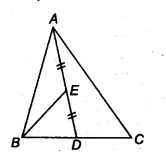Q

# In a triangle ABC, E is the mid-point of median AD. Show that ar (BED) = 1/4 ar(ABC).

Q : 2      In a triangle ABC, E is the mid-point of median AD. Show that     .

Views

We have a triangle ABC and  AD is a median. Join B and E.Since the median divides the triangle into two triangles of equal area.
ar(ABD) = ar (ACD) = 1/2 ar(ABC)..............(i)
Now, in triangle ABD,
BE is the median [since E is the midpoint of AD]
ar (BED) = 1/2 ar(ABD)........(ii)

From eq (i) and eq (ii), we get

ar (BED) = 1/2 . (1/2 ar(ar (ABC))
ar (BED)  = 1/4 .ar(ABC)

Hence proved.

Exams
Articles
Questions